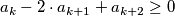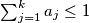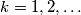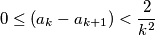### IMO Shortlist 1988 problem 24

Kvaliteta:
Avg: 0,0
Težina:
Avg: 0,0
Let$\{a_k\}^{\infty}_1$ be a sequence of non-negative real numbers such that:and$\sum^k_{j = 1} a_j \leq 1$ for all$k = 1,2, \ldots$. Prove that:for all$k = 1,2, \ldots$.
Izvor: Međunarodna matematička olimpijada, shortlist 1988